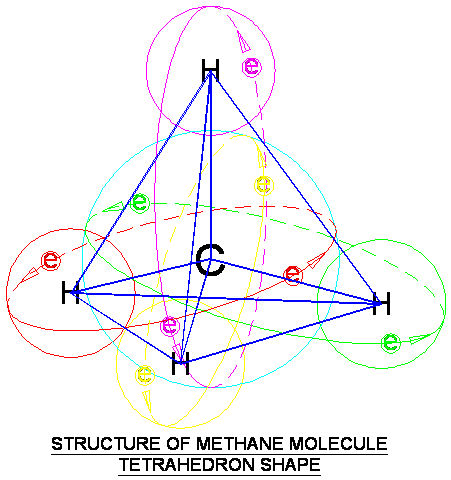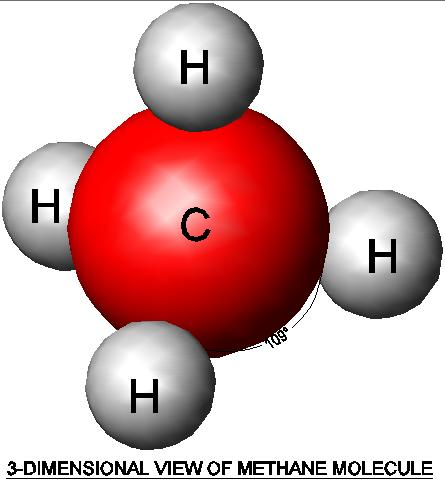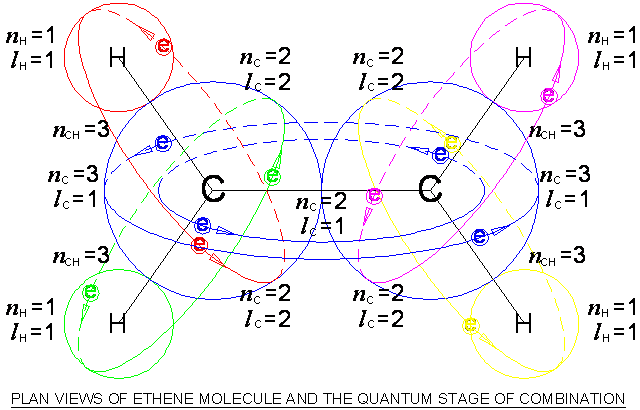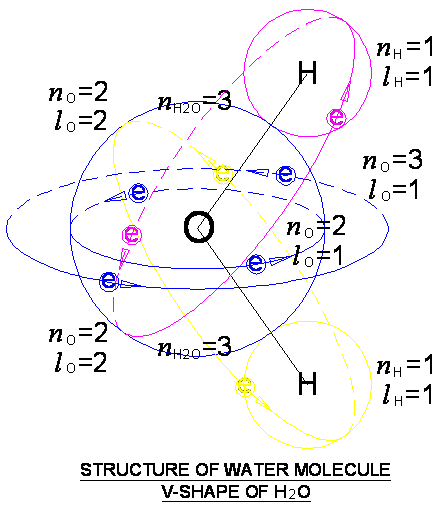PHYSICS

Mass

MASS

MA. Fundamental of Mass MB. Kong Frequency and Kong Wavelength MC. Annihilation and Pair Production

MD. Kong Equation

ME. Kong Atom Model MF. Quantum of Atom MG. Perturbation of Photon

MH. Periodic Table

MI. Chemical Reaction MJ. Superconductor MK. Particles and Waves ML. Nuclear Physics

MI. CHEMICAL REACTION

1. Ionic Bonding 2. Methane Molecule 3. Ethene Molecule

INTRODUCTION

In all the previous chapters, from “Kong Equation” until the “Periodic Table”, they describe about atoms. In this chapter, we describe about substance bigger than atom, which is the molecule. Molecule is made up of the combination of 2 atoms or more. Due to the Kong Atom Model, molecule is able to produce all kinds of structures such as tetrahedron and etcetera. Few molecule examples together with the related quantum mechanics are presented here.

From chapter "Life of Universe", it described that atoms possess reactive magnetic gauss line while electrons and positrons do not possess such property. Therefore, atoms are able to perform chemical reaction by forming molecules and energy is released. Electrons are just living in atoms or molecules after chemical reaction.

OBJECTIVES

1)      To describe and to model the structure of ionic bonding and molecule.

2)      To describe the structure of salt, methane, ethene and water molecules.

MI.1.0        IONIC BONDING

 3 Li Description n = 2, 1 Lithium has PON = 2 and 1 l = 1, 1 Electrons orbit at angle 90º for both PON Ne = 1, 2 Orbit PON=1 and SON=1 is fully filled with two electrons, the third electron fill at PON=2 and SON=1

Table MI.1.1

We quote from the chapter “Quantum of Atom”, table MI.1.1 shows the electron addresses of Lithium atom. Lithium undergoes chemical reaction by sharing one electron with another substance to achieve higher stability. For example, Lithium Chloride (LiCl), Lithium (Li) atom bond to Chlorine (Cl) atom to form LiCl salt by donating one electron to Cl. In the crystal structure of LiCl, the PON 2 of Lithium and the outest orbit of Cl are combined to form a bigger orbit so that two electrons circulate on the combined orbit. If the forming of combined orbit release energy, this process is called exothermic reaction. Before chemical reaction happen, the energy required on breaking the orbit is the activating energy.

If the ionic bonding forms salt which is soluble into water, the substance is considered as ionic bonding. In ionic bonding, ions are surrounded by water molecules. The hydrated cations and anions attract to each due to electrostatic force. The salt is soluble because the water molecule is able to reduce the charge of ionic substance and enhance the stability. If the hydration process releases heat, it is called exothermic reaction. If the hydration process absorbs heat, it is called endothermic reaction.

If the ionic bonding forms crystal which is not soluble, the bonding between atoms is more likely to covalent bonding. In covalent bonding, the orbital of each atom are combined to form bigger orbital.

MI.2.0        METHANE MOLECULE

All chemical reactions undergo the same process. Each chemical bonding causes the breaking and forming of orbit to a stable orbit and each orbit are circulated by two electrons. Due to this phenomenon, the molecule structure is able to produce all kinds of shape. For example, carbon (C) of methane (CH4) bonds with four hydrogen (H) atoms through 4 different orbits with two electrons at each orbit. Due to the 4 individual independent bonding, the hydrogen atom can freely arrange in the structure to achieve the best stability. The repulsive force between the 4 pairs of electrons and 4 number of hydrogen atoms produces the tetrahedron shape of methane.

Figure MI.2.1 suggests the methane structure. Hydrogen and carbon are combined to form 4 different orbits. Each orbit is circulated by 2 electrons. The repulsive force of 4 pairs of electrons causes the structure to self-arrange and produce the shape of tetrahedron.Figure MI.2.1

Figure MI.2.2 shows the suggested solid structure of methane. Methane possesses tetrahedron shape. The angle between each bonding is 109°.Figure MI.2.2Figure MI.2.3

Figure MI.2.3 shows two views of methane structure and the suggested quantum number after combination. The combined orbit achieves higher PON. Hydrogen atom forms bonding at PON 1 and SON 1. Carbon atom forms bonding at PON 2 and 3, SON 1 and 2. Note that the carbon and hydrogen atoms both achieve the electron addresses of Helium and Neon respectively. The PON 3 and SON 2 of combined orbital of CH4 allow the hydrogen to arrange in wide angle. Both carbon and hydrogen atoms achieve the stability with completion of electron valence.

MI.3.0        ETHENE MOLECULE

Figure MI.3.1 shows the suggested ethene molecule and its quantum number of each bonding. Molecule ethene has double bonds between two carbon atoms and another two bonds to hydrogen atom. The two carbon atoms are horizontal dual bonded and has the PON of 2 and 3 and SON of 1. When the double bond is attacked by another substance such as hydrogen atom under correct environment, the quantum number of PON 3 and SON 1 is broken, remain the bonding at PON 2 and SON 1. Two hydrogen atoms react with the double bond and produce ethane molecule. The reaction is suggested as follows,

H2C=CH2 + H–H à H3C–CH2· + H·                                               … eq. MI.3.1

H3C–CH2· + H· à H3C–CH3                                                           … eq. MI.3.2

where H3C–CH2· and H· are suggested non-stable intermediate substances.

Note that hydrogen atom achieves the electron addresses of Helium and carbon atom achieves the configuration of Neon.Figure MI.3.1

MI.4.0        WATER MOLECULE

Similar chemical reaction happened to water molecule, H2O. Figure MI.4.1 suggests the water molecule structure and the ‘V’ shape structure. Two hydrogen atoms react with oxygen atom at quantum number PON 3 and SON 2, produce the ‘V’ shape of molecule. The ‘V’ shape of water molecule possesses magnetic dipole moment. Note that hydrogen atom achieves the electron addresses of Helium, and oxygen atom achieves the configuration of Neon.Figure MI.4.1

DISCUSSIONS AND CONCLUSIONS

All chemical reactions undergo the same bonding mechanism, which is breaking of base orbit and forming combined orbit. The breaking of orbit requires energy which is the activating energy. The forming of combined orbit releases heat to achieve better stability.

Due to the Kong Atom Model, the formation of all kinds of molecule structure such as tetrahedron of methane and ‘V’ shape of water molecule is explainable and can be modeled.

This website is originated on 15-Mar-2007,

updated on 4-Jan-2009.